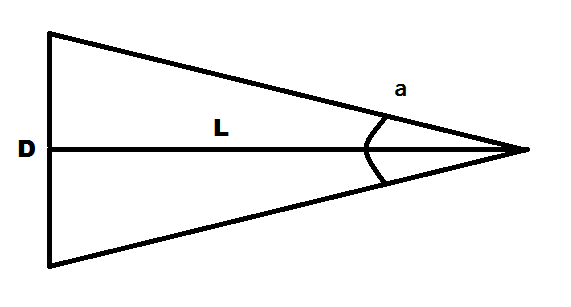homechevron_rightStudychevron_rightMathchevron_rightGeometry

# Angular size, linear size, and distance

These calculators computes value of angular size or linear size or distance from the other two'sThe angular size (angular diameter, apparent size) of an object seen from a given point is the object's visual diameter measured as an angle. The visual diameter is the diameter of the object's perspective projection on a plane through its center that is perpendicular to the viewing direction. Look at the picture.

The angular size, linear size and distance can be calculated using the formulas:

Thus, we can determine the distance from the object if we knew its size and angular size. Binoculars often have special marks, which helps to find out the angular size of the observed object.

Also, we can find out object size from its angular size and distance from it. And, of course, its angular size from its linear size and distance. This is a commonplace way of measuring things in astronomy. In astronomy, the dimensions of objects in the sky are often given in terms of their angular diameter as seen from Earth, rather than their actual sizes.

Below the calculators which can compute any parameter from two others. By default, distance from the sun to the earth, sun's diameter, and sun's average angular size are used. And, if you want to make sense of the angular size of something, be sure to check the Apparent length from angular size#### Distance from angular size and linear size

°
Digits after the decimal point: 2
Distance#### Linear size from angular size and distance

°
Digits after the decimal point: 2
Linear size#### Angular size from distance and linear size

Angular size

URL copied to clipboardPLANETCALC, Angular size, linear size, and distance